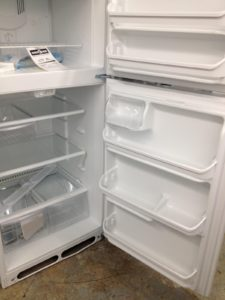# Putting It Together: Finance

##Financing a Refrigerator: Three Scenarios

In the beginning of this module, we presented three options for buying a new refrigerator. In one scenario, you could rent to own, with the following terms $17.99 per week for 2 years, which is 104 weeks. The total cost is:Scenario two involved a loan of$1299 from your brother at 20% interest for one full year.  To calculate the total amount, use the simple interest formula,In this situation, the principle amount is, rate is, and the time isyear.  Therefore, the interest due to your brother would be:Adding the interest back to the principle, the total cost of the refrigerator would amount to $1558.80. That’s quite a bit less than the$1870.96 that the rent-to-own store would ultimately have received from you.  But your brother wants the money in one year, so let’s figure out what the weekly payment would be.  Simply divide the total by 52 weeks.This is a higher weekly payment than the rent-to-own store is offering, but if you can afford it, then you’ll save money in the long run.

Finally, let’s explore the third option.  This time we use the loans formula,The principle is the same,, but the rate is now.  Because the compounding is monthly, we have. Finally,represents the total number of years for the loan.  We must solve for.This calculation gives the monthly payment (since the compounding is monthly),If we want to see how this compares against our previous scenarios, we can find an equivalent weekly payment.  The best way to do this is to multiply d by 12 and then divide by 52.  This gives a weekly payment of about, by far the lowest weekly payment, but what is the total cost?

Finally, to calculate the total cost, multiply the monthly payment by the number of months in 3 years, that is, 36 months.Option three, the line of store credit. This option seemed pretty good at first. However, because of the long loan period and compounding interest, the total cost is actually more than the $1558.80 from scenario two. Let’s compare the details of each scenario shown in the table below. Note, the total interest is found by subtracting the list price of the refrigerator ($1299) from the total paid amount.

 Rent to Own Brother’s Offer Store Loan Payments $17.99 per week$29.98 per week $45.03 per month ($10.39 per week) Length of Term 2 years 1 year 3 years Total Paid $1870.96$1558.80 $1621.09 Total Interest$571.96 $259.80$322.09

Which scenario would you choose?